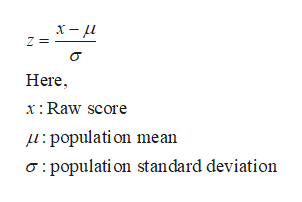# The results of a common standardized test used in psychology research is designed so that the population mean is 175 and the standard deviation is 40. A subject earns a score of 159. What is the z-score for this raw score?z-score =

Question
1 views

The results of a common standardized test used in psychology research is designed so that the population mean is 175 and the standard deviation is 40. A subject earns a score of 159. What is the z-score for this raw score?

z-score =

check_circle

Step 1

Formula for z-scor...help_outlineImage Transcriptioncloseх— и Z Here x: Raw score :population mean a: populati on stan dard deviation fullscreen

### Want to see the full answer?

See Solution

#### Want to see this answer and more?

Solutions are written by subject experts who are available 24/7. Questions are typically answered within 1 hour.*

See Solution
*Response times may vary by subject and question.
Tagged in

### Other